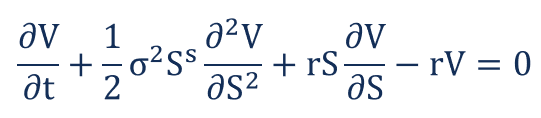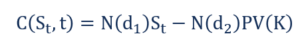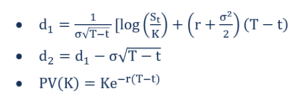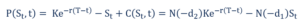# Black-Scholes-Merton Model

A pricing model used to determine the fair prices of stock options based on six variables

## What is the Black-Scholes-Merton Model?

The Black-Scholes-Merton (BSM) model is a pricing model for financial instruments. It is used for the valuation of stock options. The BSM model is used to determine the fair prices of stock options based on six variables: volatility, type, underlying stock price, strike price, time, and risk-free rate. It is based on the principle of hedging and focuses on eliminating risks associated with the volatility of underlying assets and stock options.

### The Black-Scholes-Merton Equation

The Black-Scholes-Merton model can be described as a second order partial differential equation.The equation describes the price of stock options over time.

### Pricing a Call Option

The price of a call option C is given by the following formula:Where:### Pricing a Put Option

The price of a put option P is given by the following formula:Where:

• N – Cumulative distribution function of the standard normal distribution. It represents a standard normal distribution with mean = 0 and standard deviation = 1
• T-t – Time to maturity (in years)
• St – Spot price of the underlying asset
• K – Strike price
• r – Risk-free rate
• Ó – Volatility of returns of the underlying asset

### Assumptions of the Black-Scholes-Merton Model

• Lognormal distribution: The Black-Scholes-Merton model assumes that stock prices follow a lognormal distribution based on the principle that asset prices cannot take a negative value; they are bounded by zero.
• No dividends: The BSM model assumes that the stocks do not pay any dividends or returns.
• Expiration date: The model assumes that the options can only be exercised on its expiration or maturity date. Hence, it does not accurately price American options. It is extensively used in the European options market.
• Random walk: The stock market is a highly volatile one, and hence, a state of random walk is assumed as the market direction can never truly be predicted.
• Frictionless market: No transaction costs, including commission and brokerage, is assumed in the BSM model.
• Risk-free interest rate: The interest rates are assumed to be constant, hence making the underlying asset a risk-free one.
• Normal distribution: Stock returns are normally distributed. It implies that the volatility of the market is constant over time.
• No arbitrage: There is no arbitrage. It avoids the opportunity of making a riskless profit.

#### Limitations of the Black-Scholes-Merton Model

• Limited to the European market: As mentioned earlier, the Black-Scholes-Merton model is an accurate determinant of European option prices. It does not accurately value stock options in the US. It is because it assumes that options can only be exercised on its expiration/maturity date.
• Risk-free interest rates: The BSM model assumes constant interest rates, but it is hardly ever the reality.
• Assumption of a frictionless market: Trading generally comes with transaction costs such as brokerage fees, commission, etc. However, the Black Scholes Merton model assumes a frictionless market, which means that there are no transaction costs. It is hardly ever the reality in the trading market.
• No returns: The BSM model assumes that there are no returns associated with the stock options. There are no dividends and no interest earnings. However, it is not the case in the actual trading market. The buying and selling of options are primarily focused on the returns.

### More Resources

CFI offers the Financial Modeling & Valuation Analyst (FMVA)™ certification program for those looking to take their careers to the next level. To keep learning and advancing your career, the following resources will be helpful:

• Continuously Compounded Return
• Options: Calls and Puts
• Risk-free Rate
• Spot Price

### Corporate Finance Training

Advance your career in investment banking, private equity, FP&A, treasury, corporate development and other areas of corporate finance.

Enroll in CFI’s Finance Courses to take your career to the next level! Learn step-by-step from professional Wall Street instructors today.